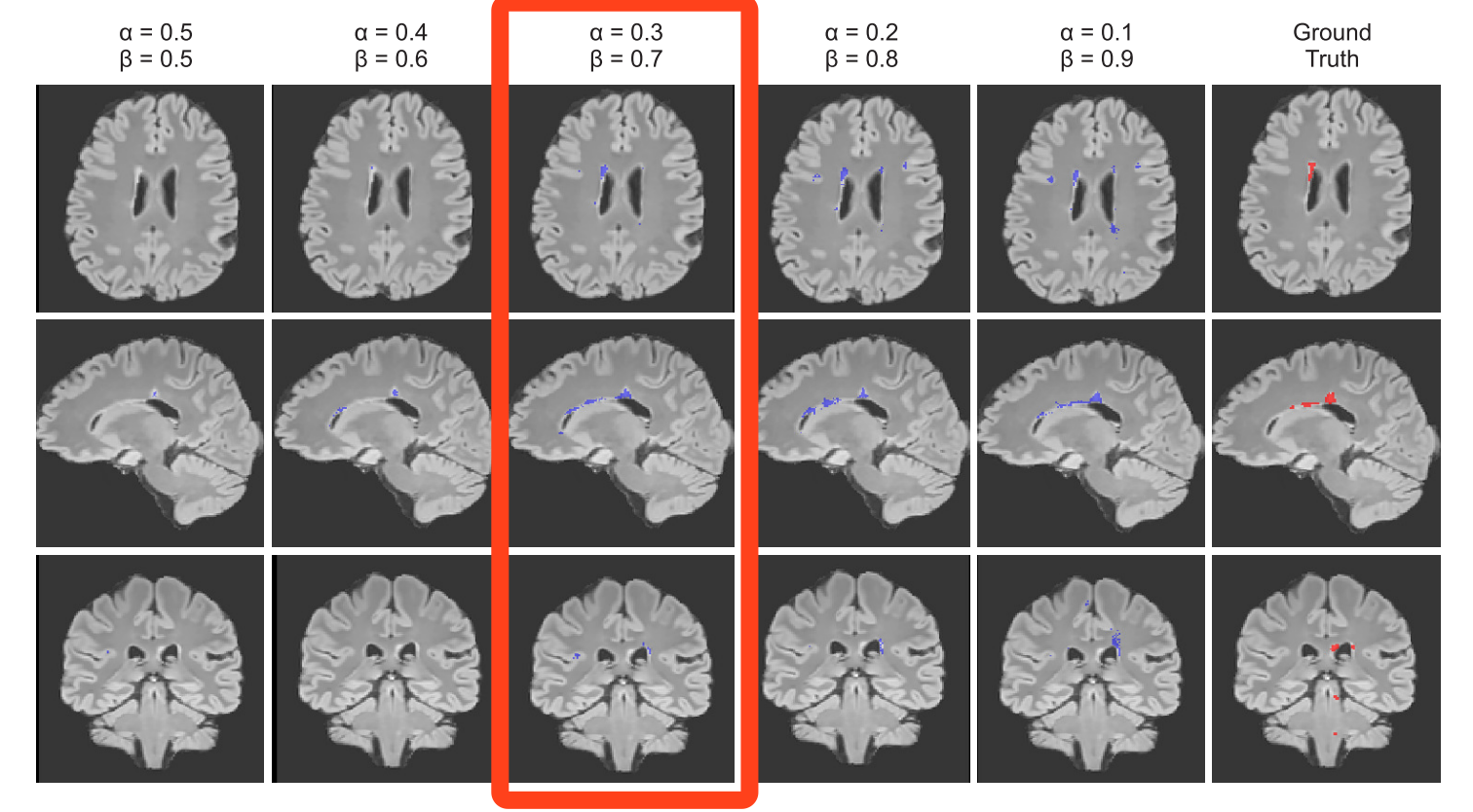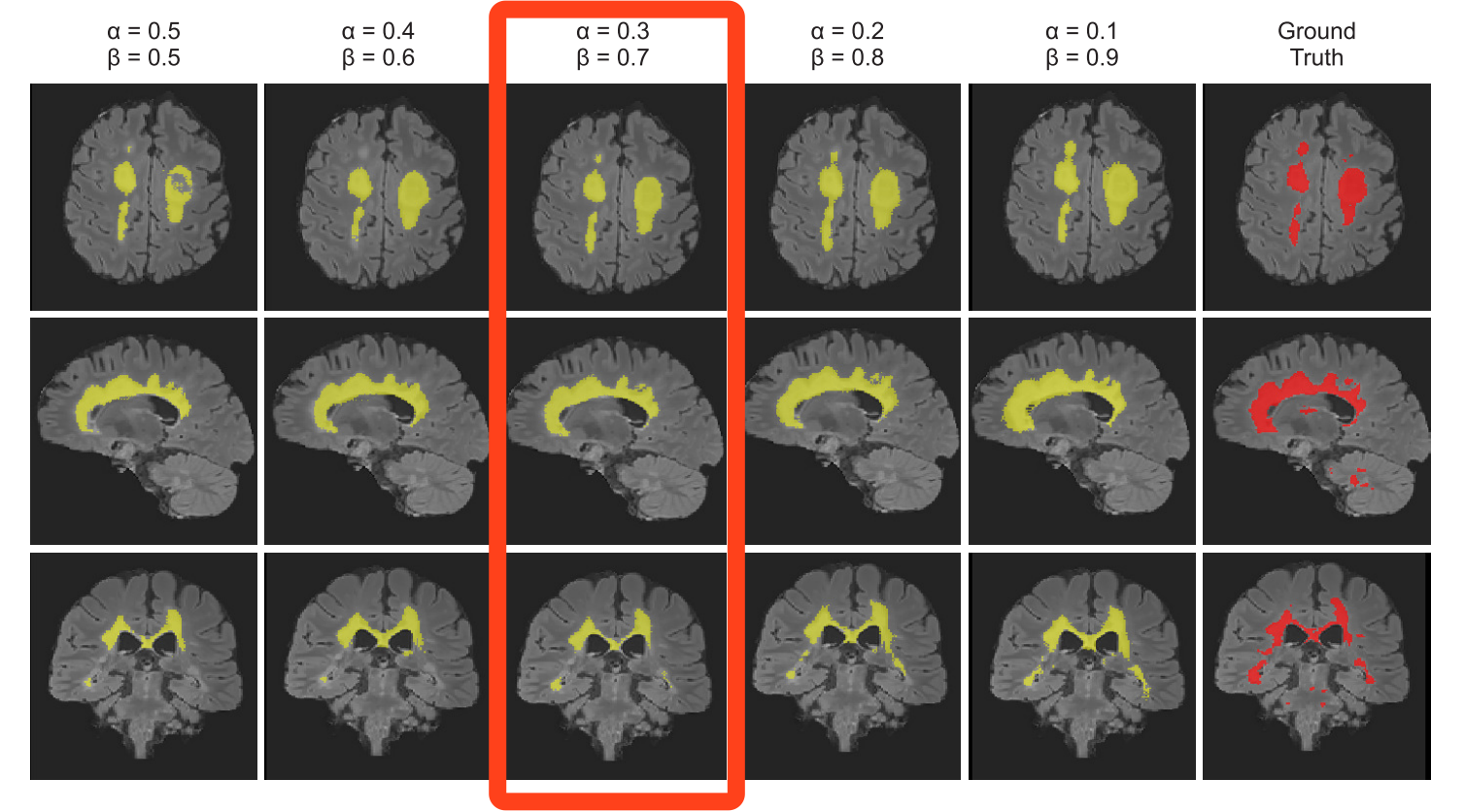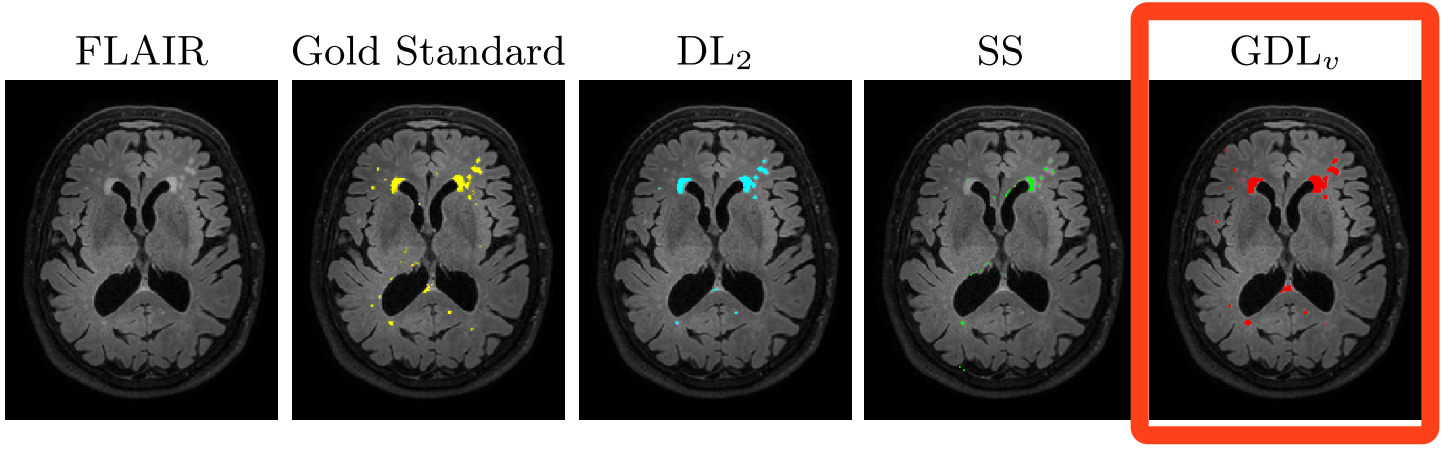• 最近在做小目标图像分割任务（医疗方向），往往一幅图像中只有一个或者两个目标，而且目标的像素比例比较小，选择合适的loss function往往可以解决这个问题。以下是我的实验比较。 场景： 1.U-Net网络 2. keras...


文章目录
前言场景loss function一、Log loss二、WCE Loss三、Focal loss四、Dice loss五、IOU loss六、Tversky loss七、敏感性--特异性 loss八、Generalized Dice loss九、BCE + Dice loss十、Dice + Focal loss十一、Exponential Logarithmic loss
参考

前言
最近在做小目标图像分割任务（医疗方向），往往一幅图像中只有一个或者两个目标，而且目标的像素比例比较小，使网络训练较为困难，一般可能有三种的解决方式：
选择合适的loss function，对网络进行合理的优化，关注较小的目标。改变网络结构，使用attention机制（类别判断作为辅助）。与2的根本原理一致，类属attention，即：先检测目标区域，裁剪之后进行分割训练。
通过使用设计合理的loss function，相比于另两种方式更加简单易行，能够保留图像所有信息的情况下进行网络优化，达到对小目标精确分割的目的。
场景
使用U-Net作为基准网络。实现使用keras小目标图像分割场景，如下图举例。 AI Challenger眼底水肿病变区域自动分割，背景占据了很大的一部分segthor医疗影像器官分割loss function
一、Log loss
对于二分类而言，对数损失函数如下公式所示：

−

1

N

∑

i

=

1

N

(

y

i

log

⁡

p

i

+

(

1

−

y

i

)

log

⁡

(

1

−

p

i

)

)

-\frac{1}{N}\sum_{i=1}^{N}(y_i\log p_i + (1-y_i)\log (1-p_i))

其中，

y

i

y_i

为输入实例

x

i

x_i

的真实类别,

p

i

p_i

为预测输入实例

x

i

x_i

属于类别 1 的概率. 对所有样本的对数损失表示对每个样本的对数损失的平均值, 对于完美的分类器, 对数损失为 0。 此loss function每一次梯度的回传对每一个类别具有相同的关注度！所以极易受到类别不平衡的影响，在图像分割领域尤其如此。 例如目标在整幅图像当中占比也就仅仅千分之一，那么在一副图像中，正样本（像素点）与父样本的比例约为1～1000，如果训练图像中还包含大量的背景图，即图像当中不包含任何的疾病像素，那么不平衡的比例将扩大到>10000，那么训练的后果将会是，网络倾向于什么也不预测！生成的mask几乎没有病灶像素区域！ 此处的案例可以参考airbus-ship-detection。
二、WCE Loss
带权重的交叉熵loss — Weighted cross-entropy (WCE) R为标准的分割图，其中

r

n

r_n

为label 分割图中的某一个像素的GT。P为预测的概率图，

p

n

p_n

为像素的预测概率值，背景像素图的概率值就为1-P。 只有两个类别的带权重的交叉熵为：

W

C

E

=

−

1

N

∑

n

=

1

N

(

w

r

n

l

o

g

(

p

n

)

+

(

1

−

r

n

)

l

o

g

(

1

−

p

n

)

)

WCE = - \frac{1}{N}\sum_{n=1}^{N}(wr_nlog(p_n) + (1 - r_n)log(1 - p_n))

w

w

为权重，

w

=

N

−

∑

n

p

n

∑

n

p

n

w=\frac{N-\sum_np_n}{\sum_np_n}

缺点是需要人为的调整困难样本的权重，增加调参难度。
三、Focal loss
能否使网络主动学习困难样本呢？ focal loss的提出是在目标检测领域，为了解决正负样本比例严重失衡的问题。是由log loss改进而来的，为了于log loss进行对比，公式如下：

−

1

N

∑

i

=

1

N

(

α

y

i

(

1

−

p

i

)

γ

log

⁡

p

i

+

(

1

−

α

)

(

1

−

y

i

)

p

i

γ

log

⁡

(

1

−

p

i

)

)

-\frac{1}{N}\sum_{i=1}^{N}(\alpha y_i(1-p_i)^{\gamma}\log p_i + (1-\alpha )(1-y_i)p_i^{\gamma}\log (1-p_i))

说白了就多了一个

(

1

−

p

i

)

γ

(1-p_i)^{\gamma}

，loss随样本概率的大小如下图所示：其基本思想就是，对于类别极度不均衡的情况下，网络如果在log loss下会倾向于只预测负样本，并且负样本的预测概率

p

i

p_i

也会非常的高，回传的梯度也很大。但是如果添加

(

1

−

p

i

)

γ

(1-p_i)^{\gamma}

则会使预测概率大的样本得到的loss变小，而预测概率小的样本，loss变得大，从而加强对正样本的关注度。 可以改善目标不均衡的现象，对此情况比 binary_crossentropy 要好很多。 目前在图像分割上只是适应于二分类。 代码：https://github.com/mkocabas/focal-loss-keras
from keras import backend as K
'''
Compatible with tensorflow backend
'''
def focal_loss(gamma=2., alpha=.25):
def focal_loss_fixed(y_true, y_pred):
pt_1 = tf.where(tf.equal(y_true, 1), y_pred, tf.ones_like(y_pred))
pt_0 = tf.where(tf.equal(y_true, 0), y_pred, tf.zeros_like(y_pred))
return -K.sum(alpha * K.pow(1. - pt_1, gamma) * K.log(pt_1))-K.sum((1-alpha) * K.pow( pt_0, gamma) * K.log(1. - pt_0))
return focal_loss_fixed

使用方法：
model_prn.compile(optimizer=optimizer, loss=[focal_loss(alpha=.25, gamma=2)])

目前实验得到结论：
经过测试，发现使用focal loss很容易就会过拟合？？且效果一般。。。I don’t know why?此方法代码有待改进，因为此处使用的网络为U-net，输入和输出都是一张图！直接使用会导致loss的值非常的大！需要添加额外的两个全局参数alpha和gamma，对于调参不方便。

以上的方法Log loss，WBE Loss，Focal loss都是从本源上即从像素上来对网络进行优化。针对的都是像素的分类正确与否。有时并不能在评测指标上DICE上得到较好的结果。

---- 更新2020-4-1 将K.sum改为K.mean, 与其他的keras中自定义的损失函数保持一致:
-K.mean(alpha * K.pow(1. - pt_1, gamma) * K.log(pt_1)) - K.mean((1 - alpha) * K.pow(pt_0, gamma) * K.log(1. - pt_0))


效果还未尝试，如果有同学尝试了可以留言交流~

四、Dice loss
dice loss 的提出是在Ｖ-net中，其中的一段原因描述是在感兴趣的解剖结构仅占据扫描的非常小的区域，从而使学习过程陷入损失函数的局部最小值。所以要加大前景区域的权重。
Dice 可以理解为是两个轮廓区域的相似程度，用A、B表示两个轮廓区域所包含的点集，定义为：

D

S

C

(

A

,

B

)

=

2

∣

A

⋂

B

∣

∣

A

∣

+

∣

B

∣

DSC(A,B) = 2\frac{|A\bigcap B |}{|A| + |B|}

其次Dice也可以表示为：

D

S

C

=

2

T

P

2

T

P

+

F

N

+

F

P

DSC = \frac{2TP}{2TP+FN+FP}

其中TP，FP，FN分别是真阳性、假阳性、假阴性的个数。 二分类dice loss:

D

L

2

=

1

−

∑

n

=

1

N

p

n

r

n

+

ϵ

∑

n

=

1

N

p

n

+

r

n

+

ϵ

−

∑

n

=

1

N

(

1

−

p

n

)

(

1

−

r

n

)

+

ϵ

∑

n

=

1

N

2

−

p

n

−

r

n

+

ϵ

DL_2 = 1 - \frac{\sum_{n=1}^{N}p_nr_n + \epsilon}{\sum_{n=1}^{N}p_n + r_n + \epsilon} -\frac{\sum_{n=1}^{N}(1 - p_n)(1 - r_n) + \epsilon}{\sum_{n=1}^{N}2 - p_n - r_n + \epsilon}

代码：
def dice_coef(y_true, y_pred, smooth=1):
intersection = K.sum(y_true * y_pred, axis=[1,2,3])
union = K.sum(y_true, axis=[1,2,3]) + K.sum(y_pred, axis=[1,2,3])
return K.mean( (2. * intersection + smooth) / (union + smooth), axis=0)
def dice_coef_loss(y_true, y_pred):
1 - dice_coef(y_true, y_pred, smooth=1)

结论：
有时使用dice loss会使训练曲线有时不可信，而且dice loss好的模型并不一定在其他的评价标准上效果更好，例如mean surface distance 或者是Hausdorff surface distance。 不可信的原因是梯度，对于softmax或者是log loss其梯度简化而言为

p

−

t

p-t

，

t

t

为目标值，

p

p

为预测值。而dice loss为

2

t

2

(

p

+

t

)

2

\frac{2t^2}{(p+t)^2}

，如果

p

p

，

t

t

过小则会导致梯度变化剧烈，导致训练困难。属于直接在评价标准上进行优化。不均衡的场景下的确好使。 论文中在前列腺MRI容积图中的分割表现：五、IOU loss
可类比DICE LOSS，也是直接针对评价标准进行优化。 在图像分割领域评价标准IOU实际上

I

O

U

=

T

P

T

P

+

F

P

+

F

N

IOU = \frac{TP}{TP + FP + FN}

，而TP，FP，FN分别是真阳性、假阳性、假阴性的个数。 而作为loss function，定义

I

O

U

=

I

(

X

)

U

(

X

)

IOU = \frac{I(X)}{U(X)}

，其中，

I

(

X

)

=

X

∗

Y

I(X) = X*Y

U

(

X

)

=

X

+

Y

−

X

∗

Y

U(X) = X + Y - X*Y

，X为预测值而Y为真实标签。
## intersection over union
def IoU(y_true, y_pred, eps=1e-6):
if np.max(y_true) == 0.0:
return IoU(1-y_true, 1-y_pred) ## empty image; calc IoU of zeros
intersection = K.sum(y_true * y_pred, axis=[1,2,3])
union = K.sum(y_true, axis=[1,2,3]) + K.sum(y_pred, axis=[1,2,3]) - intersection
return -K.mean( (intersection + eps) / (union + eps), axis=0)

在PASCAL VOC 2010上的实验效果如下，基础框架还是FCNIOU loss的缺点呢同DICE loss是相类似的，训练曲线可能并不可信，训练的过程也可能并不稳定，有时不如使用softmax loss等的曲线有直观性，通常而言softmax loss得到的loss下降曲线较为平滑。
六、Tversky loss
提到Tversky loss不得不提Tversky 系数，Tversky系数是Dice系数和 Jaccard 系数的一种广义系数，公式如下：

T

(

A

,

B

)

=

∣

A

⋂

B

∣

∣

A

⋂

B

∣

+

α

∣

A

−

B

∣

+

β

∣

B

−

A

∣

T(A,B) = \frac{|A \bigcap B|}{|A \bigcap B| + \alpha |A - B| + \beta |B - A|}

再抄一遍Dice系数公式：

D

S

C

(

A

,

B

)

=

2

∣

A

⋂

B

∣

∣

A

∣

+

∣

B

∣

DSC(A,B) = 2\frac{|A\bigcap B |}{|A| + |B|}

，此时A为预测,而B为真实标签。 观察可得当设置

α

=

β

=

0.5

\alpha = \beta = 0.5

，此时Tversky系数就是Dice系数。而当设置

α

=

β

=

1

\alpha = \beta = 1

时，此时Tversky系数就是Jaccard系数。 对于Tversky loss也是相似的形式就不重新编写了，但是在

T

(

A

,

B

)

T(A,B)

中，

∣

A

−

B

∣

|A - B|

则意味着是FP（假阳性），而

∣

B

−

A

∣

|B - A|

则意味着是FN（假阴性）；

α

和

β

\alpha和 \beta

分别控制假阴性和假阳性。通过调整

α

\alpha

和

β

\beta

我们可以控制假阳性和假阴性之间的权衡。 不同的

α

\alpha

和

β

\beta

下各个指标的结果：在极小的病灶下的分割效果图如下：在较大病灶下的分割结果：考虑到处理数据类别极度不均衡情况下的指标FP和FN，在牺牲一定精度的情况下，从而提高像素分类的召回率。
代码参考keras
def tversky(y_true, y_pred):
y_true_pos = K.flatten(y_true)
y_pred_pos = K.flatten(y_pred)
true_pos = K.sum(y_true_pos * y_pred_pos)
false_neg = K.sum(y_true_pos * (1-y_pred_pos))
false_pos = K.sum((1-y_true_pos)*y_pred_pos)
alpha = 0.7
return (true_pos + smooth)/(true_pos + alpha*false_neg + (1-alpha)*false_pos + smooth)
def tversky_loss(y_true, y_pred):
return 1 - tversky(y_true,y_pred)
def focal_tversky(y_true,y_pred):
pt_1 = tversky(y_true, y_pred)
gamma = 0.75
return K.pow((1-pt_1), gamma)

七、敏感性–特异性 loss
首先敏感性就是召回率，检测出确实有病的能力：

S

e

n

s

i

t

i

v

i

t

y

=

T

P

T

P

+

F

N

Sensitivity = \frac{TP}{TP+FN}

特异性，检测出确实没病的能力：

S

p

e

c

i

f

i

c

i

t

y

=

T

N

T

N

+

F

P

Specificity = \frac{TN}{TN+FP}

Sensitivity - Speciﬁcity (SS)提出是在:

S

S

=

λ

∑

n

=

1

N

(

r

n

−

p

n

)

2

r

n

∑

n

=

1

N

r

n

+

ϵ

+

(

1

−

λ

)

∑

n

=

1

N

(

r

n

−

p

n

)

2

(

1

−

r

n

)

∑

n

=

1

N

(

1

−

r

n

)

+

ϵ

SS = \lambda\frac{\sum_{n=1}^{N}(r_n - p_n)^2r_n}{\sum_{n=1}^{N}r_n + \epsilon}　+ (1 - \lambda)\frac{\sum_{n=1}^{N}(r_n - p_n)^2(1 - r_n)}{\sum_{n=1}^{N}(1 - r_n) + \epsilon}

其中左边为病灶像素的错误率即，

1

−

S

e

n

s

i

t

i

v

i

t

y

1- Sensitivity

，而不是正确率，所以设置λ 为0.05。其中

(

r

n

−

p

n

)

2

(r_n - p_n)^2

是为了得到平滑的梯度。
八、Generalized Dice loss
区域大小和Dice分数之间的相关性： 在使用DICE loss时，对小目标是十分不利的，因为在只有前景和背景的情况下，小目标一旦有部分像素预测错误，那么就会导致Dice大幅度的变动，从而导致梯度变化剧烈，训练不稳定。 首先Generalized Dice loss的提出是源于Generalized Dice index。当病灶分割有多个区域时，一般针对每一类都会有一个DICE，而Generalized Dice index将多个类别的dice进行整合，使用一个指标对分割结果进行量化。
GDL(the generalized Dice loss)公式如下(标签数量为2)：

G

D

L

=

1

−

2

∑

l

=

1

2

w

l

∑

n

r

l

n

p

l

n

∑

l

=

1

2

w

l

∑

n

r

l

n

+

p

l

n

GDL = 1 - 2\frac{\sum_{l=1}^{2}w_l\sum_nr_{ln}p_{ln}}{\sum_{l=1}^{2}w_l\sum_nr_{ln} + p_{ln}}

其中

r

l

n

r_{ln}

为类别l在第n个像素的标准值(GT)，而

p

l

n

p_{ln}

为相应的预测概率值。此处最关键的是

w

l

w_l

，为每个类别的权重。其中

w

l

=

1

(

∑

n

=

1

N

r

l

n

)

2

w_l = \frac{1}{(\sum_{n=1}^{N}r_{ln})^2}

，这样，GDL就能平衡病灶区域和Dice系数之间的平衡。 论文中的一个效果：但是在AnatomyNet中提到GDL面对极度不均衡的情况下，训练的稳定性仍然不能保证。 参考代码：
def generalized_dice_coeff(y_true, y_pred):
Ncl = y_pred.shape[-1]
w = K.zeros(shape=(Ncl,))
w = K.sum(y_true, axis=(0,1,2))
w = 1/(w**2+0.000001)
# Compute gen dice coef:
numerator = y_true*y_pred
numerator = w*K.sum(numerator,(0,1,2,3))
numerator = K.sum(numerator)
denominator = y_true+y_pred
denominator = w*K.sum(denominator,(0,1,2,3))
denominator = K.sum(denominator)
gen_dice_coef = 2*numerator/denominator
return gen_dice_coef
def generalized_dice_loss(y_true, y_pred):
return 1 - generalized_dice_coeff(y_true, y_pred)


以上本质上都是根据评测标准设计的loss function，有时候普遍会受到目标太小的影响，导致训练的不稳定；对比可知，直接使用log loss等的loss曲线一般都是相比较光滑的。

九、BCE + Dice loss
BCE : Binary Cross Entropy 说白了，添加二分类交叉熵损失函数。在数据较为平衡的情况下有改善作用，但是在数据极度不均衡的情况下，交叉熵损失会在几个训练之后远小于Dice 损失，效果会损失。 代码：
import keras.backend as K
from keras.losses import binary_crossentropy
def dice_coef(y_true, y_pred, smooth=1):
intersection = K.sum(y_true * y_pred, axis=[1,2,3]) ##y_true与y_pred都是矩阵！（Unet）
union = K.sum(y_true, axis=[1,2,3]) + K.sum(y_pred, axis=[1,2,3])
return K.mean( (2. * intersection + smooth) / (union + smooth), axis=0)
def dice_p_bce(in_gt, in_pred):
return 1e-3*binary_crossentropy(in_gt, in_pred) - dice_coef(in_gt, in_pred)

-------更新2018-11-8
keras.losses.binary_crossentropy

只沿最后一个轴进行计算。因此，binary_crossentropy（y_true，y_pred）+ dice_loss（y_true，y_pred）仍将是（batch_size，height，width）张量。 改进：
K.mean（binary_crossentropy（y_true，y_pred））+ dice_loss（y_true，y_pred）

正确性有待验证。

思考：Dice + Focal loss ??? ------------------更新2018-11-18

十、Dice + Focal loss
最近腾讯医疗AI新突破：提出器官神经网络，全自动辅助头颈放疗规划 | 论文 中提出了Dice + Focal loss来处理小器官的分割问题。在前面的讨论也提到过，直接使用Dice会使训练的稳定性降低，而此处再添加上Focal loss这个神器。 首先根据论文的公式：

T

P

p

(

c

)

=

∑

n

=

1

N

p

n

(

c

)

g

n

(

c

)

TP_p(c) = \sum_{n=1}^{N}p_n(c)g_n(c)

F

N

p

(

c

)

=

∑

n

=

1

N

(

1

−

p

n

(

c

)

)

g

n

(

c

)

FN_p(c) = \sum_{n=1}^{N}(1-p_n(c))g_n(c)

F

P

n

(

c

)

=

∑

n

=

1

N

p

n

(

c

)

(

1

−

g

n

(

c

)

)

FP_n(c) = \sum_{n=1}^{N}p_n(c)(1-g_n(c))

L

D

i

c

e

=

∑

c

=

0

C

T

P

n

(

c

)

T

P

p

(

c

)

+

α

F

N

p

(

c

)

+

β

F

P

p

(

c

)

−

L_{Dice} = \sum_{c=0}^{C} \frac{TP_n(c)}{TP_p(c) + \alpha FN_p(c) + \beta FP_p(c)} -

其中

T

P

p

(

c

)

，

F

N

p

(

c

)

，

F

P

p

(

c

)

TP_p(c) ，FN_p(c) ，FP_p(c)

，分别对于类别c的真阳性，假阴性，假阳性。此处的

α

=

β

=

0.5

\alpha = \beta = 0.5

，此时Tversky系数就是Dice系数，为Dice loss。 最终的loss为：

L

=

L

D

i

c

e

+

λ

L

F

o

c

a

l

=

C

−

∑

c

=

0

C

T

P

n

(

c

)

T

P

p

(

c

)

+

α

F

N

p

(

c

)

+

β

F

P

p

(

c

)

−

λ

1

N

∑

c

=

0

C

∑

n

=

1

N

g

n

(

c

)

(

1

−

p

n

(

c

)

)

2

l

o

g

(

p

n

(

c

)

)

L = L_{Dice} + \lambda L_{Focal} = C - \sum_{c=0}^{C} \frac{TP_n(c)}{TP_p(c) + \alpha FN_p(c) + \beta FP_p(c)} - \lambda \frac{1}{N} \sum_{c=0}^{C} \sum_{n=1}^{N}g_n(c)(1-p_n(c))^2log(p_n(c))

论文中头颈部癌症放疗靶区自动勾画效果展示：项目地址

Focal loss理论上应该还是会得到很大的值？

十一、Exponential Logarithmic loss
与加权的DICE LOSS进行对比，此方法觉得而且这种情况跟不同标签之间的相对尺寸无关，但是可以通过标签频率来进行平衡。 结合了focal loss以及Dice loss。此loss的公式如下：

L

E

X

P

=

w

d

i

c

e

∗

L

D

i

c

e

+

w

C

r

o

s

s

∗

L

C

r

o

s

s

L_{EXP} = w_{dice}*L_{Dice} + w_{Cross}*L_{Cross}

L

D

i

c

e

L_{Dice}

为 指数log Dice损失，

L

C

r

o

s

s

L_{Cross}

为指数交叉熵损失。

L

D

i

c

e

=

E

[

(

−

l

n

(

D

i

c

e

i

)

)

γ

D

i

c

e

]

L_{Dice} = E[(-ln({Dice}_i))^{\gamma_{Dice}}]

i

i

为label。 复习一下交叉熵损失：

L

C

E

=

−

l

o

g

(

p

l

(

x

)

)

L_{CE} = -log(p_l(x))

新的指数交叉熵损失:

L

C

r

o

s

s

=

w

l

(

−

l

n

(

p

l

(

x

)

)

)

γ

C

r

o

s

s

L_{Cross} = w_l(-ln(p_l(x)))^{\gamma_{Cross}}

其中，

w

l

=

(

∑

k

f

k

f

l

)

0.5

w_l = (\frac{\sum_kf_k}{f_l})^{0.5}

，

f

k

f_k

为标签k的出现频率，

w

l

w_l

这个参数可以减小出现频率较高的类别权重。由公式可知，

w

l

w_l

随

f

l

f_l

的增大而变小，即减小权重，反之如果

f

l

f_l

太大，那么权重会变小

γ

D

i

c

e

\gamma_{Dice}

和

γ

C

r

o

s

s

\gamma_{Cross}

，提升非线性的作用。 如下图显示的是不同的指数log非线性表现：新增添了4个参数权重分别是

w

D

i

c

e

w_{Dice}

和

w

C

r

o

s

s

w_{Cross}

，

γ

D

i

c

e

\gamma_{Dice}

和

γ

C

r

o

s

s

\gamma_{Cross}

，给调参带来不小的麻烦。

以上都是针对多种loss的简单结合或者是加权结合，可能也有些小的改进，但如何平衡两个或者多个loss也是偏经验的调参问题。

如果有好的方式方法可以在此讨论：小目标的图像语义分割，有什么解决类别不平衡的方法吗？ 所有代码将整理到GitHub。

参考
 C. H. Sudre, W. Li, T. Vercauteren, S. Ourselin, and M. J. Cardoso, “Generalised dice overlap as a deep learning loss function for highly unbalanced segmentations,” in Deep Learning in Medical Image Analysis and Multimodal Learning for Clinical Decision Support, 2017.  AnatomyNet: Deep Learning for Fast and Fully Automated Whole-volume Segmentation of Head and Neck Anatomy: https://arxiv.org/pdf/1808.05238.pdf  https://www.kaggle.com/c/airbus-ship-detection/discussion/70549  https://www.kaggle.com/kmader/baseline-u-net-model-part-1  小目标的图像语义分割，有什么解决类别不平衡的方法吗？:https://www.zhihu.com/question/297255242/answer/505965855  Generalised Dice overlap as a deep learning loss function for highly unbalanced segmentations-2017  Ronneberger, O., Fischer, P., Brox, T.: U-net: Convolutional networks for biomedical image segmentation. In: MICCAI. pp. 234–241. Springer (2015)  Brosch, T., Yoo, Y., Tang, L.Y., Li, D.K., Traboulsee, A., Tam, R.: Deep convolutional encoder networks for multiple sclerosis lesion segmentation. In: MICCAI 2015. pp. 3–11. Springer (2015) 3D Segmentation with Exponential LogarithmicLoss for Highly Unbalanced Object Sizes-MICCAI2018【论文理解】 Dice-coefficient loss function vs cross-entropy Md Atiqur Rahman and Yang Wang, Optimizing Intersection-Over-Union in Deep Neural Networks for Image Segmentation Crum, W., Camara, O., Hill, D.: Generalized Overlap Measures for Evaluation and Validation in Medical Image Analysis. IEEE TMI 25(11), 1451–1461 (nov 2006)
展开全文keras dice loss focal loss
• ## 阈值分割

千次阅读 2015-09-01 12:05:05
通过迭代法来求出分割的最佳阈值，具有一定的自适应性，这种方法适用于物体的和背景有较大的区分，即其直方图有相当清楚的波谷；具体算法步骤如下： 1.为全局阈值选择一个初始估计值T。 2.用T分割图像。产生两组...
一、迭代法  通过迭代法来求出分割的最佳阈值，具有一定的自适应性，这种方法适用于物体的和背景有较大的区分，即其直方图有相当清楚的波谷；
具体算法步骤如下：  1.为全局阈值选择一个初始估计值T。  2.用T分割图像。产生两组像素：G1有灰度值大于T的像素组成，G2有小于等于T像素组成。  3.计算G1和G2像素的平均灰度值m1和m2；  4.计算一个新的阈值:T = (m1 + m2) / 2;  5.重复步骤2和4,直到连续迭代中的T值间的差小于一个预定义参数为止。
实现代码：
clear;
clc;
close all;
T=0.01;
I=im2double(I);
% T1=(min(I(:))+max(I(:)))/2;
T1=mean2(I);
index=find(I<T1);
index2=find(I>=T1);
T2=(mean2(I(index))+mean2(I(index2)))/2;
count=0;
while abs(T2-T1)>T
T1=T2;
index=find(I<T1);
index2=find(I>=T1);
T2=(mean2(I(index))+mean2(I(index2)))/2;
count=count+1;
end

count
T2
figure,imhist(I)
figure,imshow(I)
I2=im2bw(I,T2);
figure,imshow(I2);
实验证明，该方法只适用于，直方图有明显波谷的情况，其他情况不理想；

二、Ostu阈值分割法（最大类间方差法）  （1）基本原理：利用最佳阈值，将图像的灰度分割成两部分，使得两部分之间的方差最大，具有最大分离性；  （2）原理解释：当背景类信息被错误的分为目标类信息，或目标类信息被错误的分为背景类信息时，都会导致目标和背景之间的差别变小。正是由于这一特性，只要使类间方差最大或类内方差最小，这样的分割就意味着最小的误差，并且根据该准则选取最佳阈值使得目标和背景之间的分离性最好。  （3）优缺点：该方法的优点是运算量不大，在一定条件下受图像对比度与亮度变化的影响很小，在一些实时图像处理系统中得到了很广泛的应用。缺点是该方法只是借助了图像的像素灰度信息，并未考虑像素间的空间相关信息（如邻域信息），加之外部干扰的存在，使得灰度直方图的波峰和波谷并不一定明显，因而不能得到满意的分割效果，抗干扰性较差。  （4）基本步骤：
计算输入图像的归一化直方图计算累积和P1(k);计算累积均值m(k);计算全局灰度均值mG;计算类间方差；类间方差=[(mG*P1(k)-m(k))^2]/[P1(k)*(1-P1(k))];得到最佳阈值，即使得类间方差最大的k值；如果最大值不唯一，则检测到各个最大值k的平均值在最优阈值处计算可分性度量;可分性度量=最大类间方差/全局方差；全局方差=sum((i-mG)^2*p(i)) （5）下面的代码为graythresh函数里面的实现部分，也可以直接利用该函数；其中T为最优阈值，SM为可分性度量
clear;
clc;
%计算直方图
h=imhist(I);
%归一化
h=h/sum(h);
%累积分布概率
P1=cumsum(h);
i=(1:numel(h))';
%直到灰度级k的均值
m=cumsum(i.*h);
%全局均值
mG=m(end);
%类间方差,加eps防止除数为零
sigma=((mG*P1-m).^2)./(P1.*(1-P1)+eps);
%最大的类间方差
sigmaMax=max(sigma);
%假设最大不止一个取均值
T=mean(find(sigma==sigmaMax));
%最优阈值归一化,减一是因为图像的索引是0-255,但是matlab索引是1-256
T=(T-1)/(numel(h)-1)
%可分性度量
SM=sigmaMax/(sum(((i-mG).^2).*h)+eps);

三、用图像平滑改善全局阈值处理  由于受到噪声的干扰，上述方法在处理图像时，会不理想，最好是先进行平滑去噪，然后在进行阈值分割
展开全文迭代法 matlab
• ## 图像分割

千次阅读 2018-10-08 14:12:33
图像分割的主要算法： 1.基于阈值的分割方法 2.基于边缘的分割方法 3.基于区域的分割方法 4.基于聚类分析的图像分割方法 5.基于小波变换的分割方法 6.基于数学形态学的分割方法 7.基于人工神经网络的分割方法...
图像分割的主要算法：
1.基于阈值的分割方法
2.基于边缘的分割方法
3.基于区域的分割方法
4.基于聚类分析的图像分割方法
5.基于小波变换的分割方法
6.基于数学形态学的分割方法
7.基于人工神经网络的分割方法
基于阈值的分割方法
阈值分割方法作为一种常见的区域并行技术，就是用一个或几个阈值将图像的灰度直方图分成几个类，认为图像中灰度值在同一类中的像素属于同一物体。由于是直接利用图像的灰度特性，因此计算方便简明、实用性强。显然，阈值分割方法的关键和难点是如何取得一个合适的阈值。而实际应用中，阈值设定易受噪声和光亮度影响。近年来的方法有：用最大相关性原则选择阈值的方法、基于图像拓扑稳定状态的方法、Yager测度极小化方法、灰度共生矩阵方法、方差法、熵法、峰值和谷值分析法等，其中，自适应阈值法、最大熵法、模糊阈值法、类间阈值法是对传统阈值法改进较成功的几种算法。更多的情况下，阈值的选择会综合运用2种或2种以上的方法，这也是图像分割发展的一个趋势。
特点
阈值分割的优点是计算简单、运算效率较高、速度快。全局阈值对于灰度相差很大的不同目标和背景能进行有效的分割。当图像的灰度差异不明显或不同目标的灰度值范围有重叠时，应采用局部阈值或动态阈值分割法。另一方面，这种方法只考虑像素本身的灰度值，一般不考虑空间特征，因而对噪声很敏感。在实际应用中，阈值法通常与其他方法结合使用。

基于边缘的分割方法
基于边缘检测的分割方法试图通过检测包含不同区域的边缘来解决分割问题，是最常用的方法之一。通常不同的区域之间的边缘上像素灰度值的变化往往比较剧烈，这是边缘检测得以实现的主要假设之一。常用灰度的一阶或者二阶微分算子进行边缘检测。常用的微分算子有一次微分(sobel算子，Robert算子等)，二次微分(拉普拉斯算子等)和模板操作(Prewit算子，Kirsch算子等)。
特点
基于边缘的分割方法其难点在于边缘检测时抗噪性和检测精度之间的矛盾。若提高检测精度，则噪声产生的伪边缘会导致不合理的轮廓；若提高抗噪性，则会产生轮廓漏检和位置偏差。为此，人们提出各种多尺度边缘检测方法，根据实际问题设计多尺度边缘信息的结合方案，以较好的兼顾抗噪性和检测精度。
基于区域的分割方法
区域分割的实质就是把具有某种相似性质的像索连通，从而构成最终的分割区域。它利用了图像的局部空间信息，可有效地克服其他方法存在的图像分割空间小连续的缺点。在此类方法中，如果从全图出发，按区域属性特征一致的准则决定每个像元的区域归属，形成区域图，常称之为区域生长的分割方法。如果从像元出发，按区域属性特征一致的准则，将属性接近的连通像元聚集为区域，则是区域增长的分割方法。若综合利用上述两种方法，就是分裂合并的方法。它是先将图像分割成很多的一致性较强的小区域，再按一定的规则将小区域融合成大区域，达到分割图像的目的。
特点
基于区域的分割方法往往会造成图像的过度分割，而单纯的基于边缘检测方法有时不能提供较好的区域结构，为此可将基于区域的方法和边缘检测的方法结合起来，发挥各自的优势以获得更好的分割效果。
基于聚类分析的图像分割方法
特征空间聚类法进行图像分割是将图像空间中的像素用对应的特征空间点表示，根据它们在特征空间的聚集对特征空间进行分割，然后将它们映射回原图像空间，得到分割结果。其中，K均值、模糊C均值聚类(FCM)算法是最常用的聚类算法。K均值算法先选K个初始类均值，然后将每个像素归入均值离它最近的类并计算新的类均值。迭代执行前面的步骤直到新旧类均值之差小于某一阈值。模糊C均值算法是在模糊数学基础上对K均值算法的推广，是通过最优化一个模糊目标函数实现聚类，它不像K均值聚类那样认为每个点只能属于某一类，而是赋予每个点一个对各类的隶属度，用隶属度更好地描述边缘像素亦此亦彼的特点，适合处理事物内在的不确定性。利用模糊C均值(FCM)非监督模糊聚类标定的特点进行图像分割，可以减少人为的干预，且较适合图像中存在不确定性和模糊性的特点。
聚类方法应注意几个问题：
(1)聚类的类数如何确定。
(2)怎样确定聚类的有效性准则。
(3)聚类中心的位置和特性事先不清楚时，如何设置初始值。
(4)运算的开销。
并且FCM算法对初始参数极为敏感，有时需要人工干预参数的初始化以接近全局最优解，提高分割速度。另外，传统FCM算法没有考虑空间信息，对噪声和灰度不均匀敏感。
基于小波变换的分割方法
基于小波变换的阈值图像分割方法的基本思想是，首先由二进小波变换将图像的直方图分解为不同层次的小波系数，然后依据给定的分割准则和小波系数选择阈值门限，最后利用阈值标出图像分割的区域。整个分割过程是从粗到细，有尺度变化来控制，即起始分割由粗略的L2(R)子空间上投影的直方图来实现，如果分割不理想，则利用直方图在精细的子空间上的小波系数逐步细化图像分割。分割算法的计算馈与图像尺寸大小呈线性变化。小波变换为信号在不同尺度上的分析和表征提供了一个精确和统一的框架。从图像分割的角度来看，小波分解提供了一个数学上完备的描述；小波变换通过选取合适的滤波器，可以极大地减少或去除所提取的不同特征之间的相关性，不仅具有“变焦”特性，而且在实现上有快速算法。
特点
小波变换是一种多尺度、多通道的分析工具它是空域和频域的局域变换，因而能有效地从信号中提取信息，通过伸缩和平移等运算功能对函数或信号进行多尺度分析，解决了傅立叶变换不能解决的许多问题。近年来多进制小波开始用于边缘检测。另外，利用正交小波基的小波变换也可提取多尺度边缘，并可通过对图像奇异度的计算和估计来区分一些边缘的类型。
基于数学形态学的分割方法
数学形态学是一种非线性滤波方法，可以用于抑制噪声、特性提取、边缘检测、图像分割等图像处理问题。数学形态学首先被用来处理二值图像，后来也被用来处理灰度图像，现在又有学者开始用软数学形态学和模糊形态学来解决计算机视觉方面的问题。数学形态学的特点是能将复杂的形状进行分解，并将有意义的形状分量从无用的信息中提取出来。它的基本思想是利用一个称为结构元素的探针来收集图像的信息，当探针在图像中不断的移动时，不仅可根据图像各个部分间的相互关系来了解图像的结构特征，而且利用数学形态学基本运算还可以构造出许多非常有效的图像处理与分析方法。其基本的形态运算是腐蚀与膨胀。腐蚀具有使目标缩小、目标内孔增大以及外部孤立噪声消除的效果;而膨胀是将图像中与目标物体接触的所有背景点合并到物体中的过程，结果是使目标增大、孔径缩小，可以增补目标中的空间，使其形成连通域。数学形态学中另一对基本运算方法是开运算和闭运算。开运算具有消除图像是细小物体，并在物体影响纤细处分离物体和平滑较大物体边界的作用;闭运算具有填充物体影像内细小空间， 接邻近物体和平滑边界的作用。
特点
数学形态学应用于图像分割，具有定位效果好、分割精度高、抗噪声性能好的特点。同时这种方法也有着自身的局限性：由于在图像处理的前期工作中，采用数学形态学的开(闭)运算，进行图像处理后，依然存在大量与目标不符的短线和孤立点;由于预处理工作的不彻底，还需要进行一系列的基于点的开(闭)运算，因此运算速度明显下降。如何将数学形态学与其它方法综合运用以克服这些缺陷，将是数学形态学以后的工作方向。连接邻近物体和平滑边界的作用。
基于人工神经网络的分割方法
近年来，人工神经网络识别技术已经引起了广泛的关注，并应用于图像分割。基于神经网络的分割方法的基本思想是通过训练多层感知机来得到线性决策函数，然后用决策函数对像素进行分类来达到分割的目的
特点
用人工神经网络的方法分割图像，需要大量的训练数据。神经网络存在巨量的连接，容易引入空间信息，能较好地解决图像中的噪声和不均匀问题。选择何种网络结构是这种方法要解决的主要问题。
基于遗传学算法的分割方法
遗传算法(GA)，是一种模拟自然选择和遗传机制的搜索和优化过程，它具有很强的全局优化搜索能力，是一种具有广泛适用性的自适应搜索方法。它在搜索空间中是在种群中而不是在单点上进行寻优，它在求解过程中使用遗传操作规则而不是确定性规则来工作。这些特点使得遗传算法很适于应用在图像分割中，尤其是阈值分割法以及区域生长法中。利用GA的全局寻优能力及对初始位置的不敏感特性，可以改进图像分割的性能。
特点

遗传算法应用于图像分割，其难点在于适应度函数的选择以及交叉概率和变异概率的确定。GA还有可能收敛于局部最优。可考虑使用能够自适应设置交叉概率和变异概率自适应遗传算法以及和模拟退火法相结合的混合遗传算法。
--------------------- 本文来自 qq_28942201 的CSDN 博客 ，全文地址请点击：https://blog.csdn.net/qq_28942201/article/details/79376188?utm_source=copy
展开全文• Boilsoft Video Splitter 是目前分割速度最快的媒体分割器，既可分割视频文件，也可分割音频文件。支持分割 *.mpg、*.mpeg、*.avi、*.div、*.m1v、*.m2v、*.mp1、*.mp2、*.mp3 等主流视频格式为一个个小片段，你可以...
• ## 单词分割

千次阅读 2014-04-18 01:16:05
给定一个字符串S，同时给定一个字典dict，判断字符串S是否可以被分割为一个个字典里面的单词，也就是判断字符串S是否有字典里面的单词链接而成的。 例如，给定：  s = “leetcode”,  dict = ["leet", "code"]. 则...
给定一个字符串S，同时给定一个字典dict，判断字符串S是否可以被分割为一个个字典里面的单词，也就是判断字符串S是否有字典里面的单词链接而成的。
例如，给定：
s = “leetcode”,       dict = ["leet", "code"].
则结果为真，因为字符串S可以分割为leet 和code两个合法单词。
1.普通方法
bool WordBreakHelper(string& str, set<string>& dict, int nStart)
{
if (nStart == str.length())
{
return true;
}

for (set<string>::iterator iter=dict.begin(); iter != dict.end(); iter++)
{
int nLen = (*iter).length();
int nEnd = nStart + nLen;

if (nEnd > str.length())
{
//单词太长直接超过了str字符串剩余部分长度。因此不可能在字符串Str中
continue;
}

if(str.substr(nStart,nLen) == *iter)
{
if (WordBreakHelper(str, dict, nStart+nLen))
{
return true;//想一想为什么这么递归
}
}
}
return false;
}时间负责度是O(n^2)。

2.动态规划的方法
用动态规划的方法来解决单词分割的关键是：
1、定义一个数组t[],t[i]==true代表字符串的前i个字符是可以用给定字典分割的。
2、数组的初始状态为t==true。

bool WordBreak(string& str, set<string>& dict)
{
bool *bAry = new bool[str.length()+1];//想一想为什么要加1
memset(bAry,false,str.length()+1);

bAry = true;

for (int i=0; i<str.length(); i++)
{
if (!bAry[i])
{
continue;
}
//想一想为什么要在以i代表的位置为立足点对所有可能的单词进行扫描（这很必要）
for (set<string>::iterator iter = dict.begin(); iter!=dict.end(); iter++)
{
int nLen = (*iter).length();
int nEnd = i+nLen;

if (nEnd>str.length())
{
continue;
}

if (bAry[nEnd])//想一想什么时候发生这种情况
{
continue;
}

if (str.substr(i,nLen) == *iter)
{
bAry[nEnd] = true;
}
}
}
return bAry[str.length()];
}
时间复杂度为O(str.length()*dict.size())。

即使是形如如下的特殊情况,该方法仍然能很好的进行判断字符串是否能被字典分割。

输入: "programcreek", ["programcree","program","creek"].  3.更多有趣的问题

动态规划的方法虽然可以判断一个字符串S是否可以被给定的字典里的单词分割，但却不能够获悉到底是分割成了什么哪些单词。那么如何解决这个问题呢？
一个可行的办法（from jk451）如下：
将数组布尔数组bAry换做整形数组nAray。
1、将bAry[nEnd)=true替换为nAry[nEnd)=i,这意味着当你找到一个0到nEnd位置的子串可分割时，你能够得到改子串分割成的最后单词是i到nEnd位置的字母所组成的单词；
2、如果断定字符串S能够分割成为字典中的单词，那么只需要检查nAry[s.length()]里面的值，分割成的最后一个单词必然是从nAry[s.length()]到s.length()-1的位置中的字母组合成的单词，重复这个过程，可以获得其它的单词。
一点补充：当然你会发现字符串S可分割情况并不是唯一的，例如，S="nihaonihao“，字典dict=｛"ni","nihao","hao"}.此时可以分成{"nihao","nihao"}、{"ni","hao","nihao"}\.......等多种情况。

展开全文字符串 字典
• 附件包括两种文件分割工具，非代码。 1.SQL文件分割工具，可将大的SQL文件分割成若干个小文件，无需安装，直接运行...可分割合并txt,sql等任意文件，但是视频文件能分割，需安装。 文件分割合并 V1.5.exe
• ## 图像分割综述

万次阅读 多人点赞 2019-07-09 22:03:48
图像分割是计算机视觉研究中的一个经典难题，已经成为图像理解领域关注的一个热点，图像分割是图像分析的第一步，是计算机视觉的基础，是图像理解的重要组成部分，同时也是图像处理中最困难的问题之一。所谓图像分割...
• 该插件提供分割上传和不分割上传2中上传方式，实现异步上传，能防止上传文件过大而导致请求超时等问题！
• 在数字图像处理中，图像分割是很关键的一步，当图像质量较好，光照很均匀的时候只需用全局阈值的方法就能很完美地完成图像分割任务，但是有些时候会遇到光照均匀的现象，这个时候就需要用一些技巧才能达...图像处理
• ## 天空分割

千次阅读 2019-01-31 21:13:14
第一步我认为最有价值，直接在原始数据判断天空不是很好做，作者观察到天空部分整体来说是比较平滑的，也就是相邻像素之间变化不大，因此用梯度来表示则更容易识别，...第四步对分割后的图的每个联通区域统计其原始...
• ## 图像分割—灰度阈值分割

万次阅读 多人点赞 2019-08-14 11:39:55
阈值分割概念图像阈值分割具有直观和易于实现的特点，在图像分割应用中占有重要地位。许多情况下，图像$f(x,y)$由暗对象和亮对象这两类具有不同灰度级的区域组成，如报纸和书本。这种图像的亮暗部分可以在直方图中...数字图像处理 阈值 背景
• 之前看过一篇使用分割思想进行目标检测，所以这里补习下一些分割相关的基础知识。...语义分割（下图左）就是需要区分到图中每一点像素点，而仅仅是矩形框框住了。但是同一物体的不同实例需要单独分...
• Opencv 图像分割： 阈值化分割 区域分割 边缘分割 卷积 就是两个函数之间的相互关系，然后得出一个新的值，在连续空间做积分计算，然后在离散空间内求和的过程。 在计算机视觉里面，可以把卷积当做一个抽象的过程...opencv
• ## 分水岭分割方法

千次阅读 2018-11-07 17:07:48
• 语义分割 图像的语义分割是将输入图像中的每个像素分配一个语义类别，以...解码器的任务是将编码器学习到的判别特征（较低分辨率）从语义上投影到像素空间（较高分辨率），以获得密集分类。 不同于分类任务中网络...
• ## OpenCV阈值分割

千次阅读 2018-11-16 11:26:56
什么是阈值？ 最简单的图像分割的方法。 应用举例：从一副图像中利用阈值分割出我们需要的物体部分（当然这里的物体可以是一部分或者整体）。这样的图像分割方法是基于图像中物体与...即：物体在不同的图像中有...
• ## 中点分割算法

千次阅读 2019-10-21 20:48:18
中点分割算法分成两个平行的过程进行，即从P1点出发找出离P1最远的可见点（图中的B点），和从P2点出发找出离P2最远的可见点（图中的A点）。这两个可见点的连线就是原线段的可见部分。 算法的基本流程： （求 ...计算机图形学 pyqt5 python
• ## Unet目标分割

千次阅读 2019-07-27 22:52:48
Unet代码试运行 本文使用的工程代码来源： ...知乎的另一个工程：利用Unet进行人体分割，宋天中： https://zhuanlan.zhihu.com/p/35791509 制作UNet数据集 https://blog.csdn.net/awyyauqpmy/article...深度学习
• 阈值分割我们曾在3.5节学习过灰度阈值变换的相关知识， 利用灰度阈值变换分割图像就称为阈值分割， 它是一种基本的图像分割方法。 阙值分割的基本思想是确定一个阈值， 然后把每个像素点的灰度值和阈值相比较，根据...技术 图像处理
• ## pytorch语义分割

万次阅读 2018-04-05 00:19:13
Pytorch语义分割最近整合了部分pytorch实现的图象语义分割模型，简单做一下总结，代码在git：pytorch-semantic-segmentation一、简介 基于深度的图象语义分割任务不同于分类与检测任务，分类任务利用深度模型提取...pytorch fcn segnet
• ## Halcon阈值分割

千次阅读 2018-12-30 10:41:38
Halcon阈值分割 1. threshold(Image : Region : MinGray, MaxGray : ) 全局阈值分割，适用于环境稳定，目标与背景存在明显的灰度差的场合。 应用1：利用灰度直方图确定阈值进行图像分割。一般是物体与背景之间...Halcon
• 对一副图像进行分块阈值，解决光照不均分割不足的问题。通过判断类间灰度差以排除纯背景或纯物体的干扰，详细原理见文章：https://blog.csdn.net/kk55guang2/article/details/78475414
• ## RecyclerView分割线

千次阅读 2016-10-18 18:12:47Recycler android 列表...